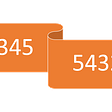# Trapping Rain Water — Day 11(Python)

Today we will be looking at one of the leetcode’s hard tagged questions.

42. Trapping Rain Water

Given n non-negative integers representing an elevation map where the width of each bar is 1, compute how much water it can trap after raining.

The above elevation map is represented by array [0,1,0,2,1,0,1,3,2,1,2,1]. In this case, 6 units of rain water (blue section) are being trapped.

Example:

`Input: [0,1,0,2,1,0,1,3,2,1,2,1]Output: 6`

Before we look into the solution, let us run through a few examples.

`Input: [3, 0, 3]Output: 3`
`Input: [3, 0, 1, 0, 3]Output: 8`
`Input: [3, 0, 1, 0, 2]Output: 5`

Considering 2 histogram bars at a time, the enclosed space between the bars is the quantity of water. Let us look at the algorithm.

1. Run the loop until all bars in the array is traversed.

2. Find the max value on the left side of the current bar. Let this value be left_max.

3. Find the max value on the right side of the current bar. Let this value be right_max.

4. Find the minimum between left_max and right_max.

5. Subtract the minimum value from the current bar.

6. If the resulting value is greater than 0, add it to our result. Since we cannot have a negative amount of water being trapped, we ignore it.

`class RainWaterTrapper:    def trap(self, height: List[int]) -> int:        total_water = 0        for i in range(1, len(height)-1):            l = max(height[:i])            r = max(height[i+1:])            water = min(l, r) - height[i]            if(water>=0):                total_water += water        return total_water`

Complexity analysis.

Time Complexity

We are traversing through the complete array which needs O(N). Additionally, we calculate max values while traversing through the array which takes O(N). Hence the time complexity is O(N²).

Space Complexity

Since we are not using any extra space, space complexity is O(1).

Can we make the algorithm any better?

We keep calculating the max value each time we hit a new bar. Can we keep track of max value beforehand? We can have 2 arrays that keep track of max value for the left side and the right side.

1. Create an array for left and create an array for right, initialize both as 0.
2. Run the loop for the left as well as the right of each bar and keep track of the max value and save them in the respective positions.
3. Once the above steps are completed, run the loop for each bar in the array.
4. Find the minimum among the left, right, and subtract it from the current bar. Include this quantity in total water.
`class RainWaterTrapper:    def trap(self, height: List[int]) -> int:        if height == []:            return 0        left = [0 for i in range(len(height))]        right = [0 for i in range(len(height))]        total_water = 0                left = height        for i in range(1, len(height)):            left[i] = max(left[i-1], height[i])                right[-1] = height[-1]        for i in range(len(height)-2, -1, -1):            right[i] = max(right[i+1], height[i])                for i in range(len(height)):             total_water += min(left[i], right[i]) - height[i]           return total_water`

Time Complexity

We are traversing through the complete array which needs O(N).

Space Complexity

We are using 2 extra arrays of size N to store max values, hence space complexity is O(N).

Kindly let me know in case you find any errors in the article. Additionally, I would like to improve my writing skills, any suggestions or comments are welcomed.

--

--

--

## More from Analytics Vidhya

Analytics Vidhya is a community of Analytics and Data Science professionals. We are building the next-gen data science ecosystem https://www.analyticsvidhya.com

## How to make VS Code Beautiful?## Information Architecture — e\$aver app## When APIs become real products## Running Docker Inside and Outside of a Jenkins Container, Along With Docker-Compose — a Tiny…## Skipping Intro Cinematic## There Is Only One Web Project## Annamariya Tharayil

Software Engineer. Find me @ www.linkedin.com/in/annamariya-jt

## Elementary operations in PyCUDA## Python Variables and Their Type## Git is not that hard to learn…## Reverse integer